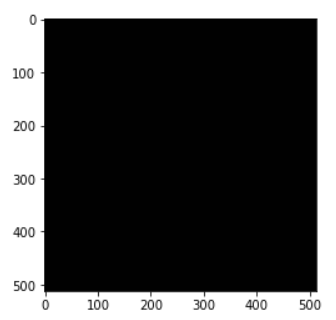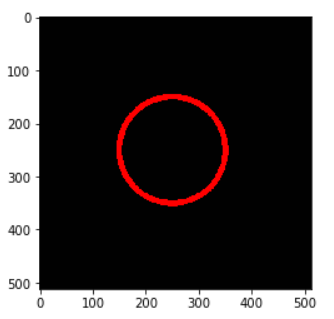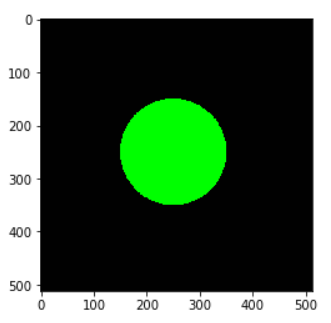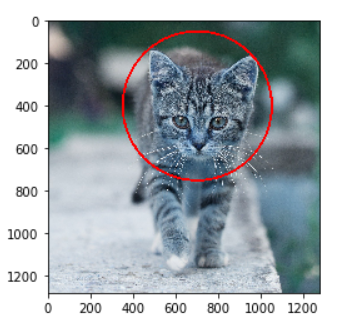# Quick Guide for Drawing Circle in OpenCV Python using cv2.circle() with Examples## Introduction

In this article, we will go through a tutorial for drawing circles in OpenCV Python by using cv2.circle() function. You may have to draw circles in OpenCV for marking an object in an image or for other creative purposes. We will explain to you cv2.circle() syntax along with few examples for a better understanding for beginners.

## Circle in OpenCV Python : cv2.circle()

We can draw circle in OpenCV python quite easily by using cv2.circle() function. Let us see the syntax of this function below –

### Syntax

• img – It is the image on which the circle has to be drawn.
• center – It is the coordinates of the center of the circle
• color – It is the color of the circle in RGB.
• thickness – It is the thickness of the circle line.

It does not return anything and draws the circle on the original image in place, this means the circle is drawn permanently on the image.

## Examples of cv2.circle() in Python OpenCV

### Import Required Libraries

Before starting the examples let us first import the required libraries as shown below –

In :

```import cv2
import numpy as np
import matplotlib.pyplot as plt

%matplotlib inline```

### Utility Function to Create Empty Image

We will create a utility function that creates an empty image of the size 512×512 and 3 color channels on which we will draw circles for examples.

In :

```def generate_empty_image():

return np.ones(shape=(512,512,3), dtype=np.int16)```

Let us see this function in action by calling it below.

In :

```sample_img = generate_empty_image()
plt.imshow(sample_img)```

Out:

<matplotlib.image.AxesImage at 0x1e14d0dc9b0>### Example – 1: Draw Simple Circle with cv2.circle()

In the first example, we will draw a simple circle on the empty image whose line is red in color and line thickness is 10.

We generate the blank image and pass it to cv2.circle() along with the center coordinates of the circle, the radius of the circle, the RGB value of red (255,0,0), and line thickness = 10.

In :

```image1 = generate_empty_image()

cv2.circle(img=image1, center = (250,250), radius =100, color =(255,0,0), thickness=10)

plt.imshow(image1)```

Out:### Example – 2: Draw Filled Circle with cv2.circle()

In this example, we are going to draw a filled circle on the blank image. The color of the fill is green.

In OpenCV to fill the circle we use the thickness = -1 in the cv2.circle() function.

In :

```image2 = generate_empty_image()

cv2.circle(img=image2, center = (250,250), radius =100, color =(0,255,0), thickness=-1)

plt.imshow(image2)```

Out

<matplotlib.image.AxesImage at 0x249bf6f1dd8>### Example – 3 : Draw Circle on Image with cv2.circle()

In this example, we will draw a circle on a cat image in red color. We have manually calculated the values of center and radius so that the circle is drawn properly on the cat’s face.

In :

```image3 = cv2.imread('cat.jpg')
cv2.circle(img=image3, center = (700,400), radius =350, color =(255,0,0), thickness=10)
plt.imshow(image3)```

Out:

<matplotlib.image.AxesImage at 0x249c2dde550>## Conclusion

Hope you found this quick tutorial on drawing circles in OpenCV quite helpful. We covered the syntax of the cv2 circle() function along with various examples for a better understanding of beginners.

Reference – OpenCV documentation